Next: The diff (and Diff) Up: Preliminaries about Maple Previous: Assignments, Functions and Constants

# The limit command

The limit command is used to compute limits. Its syntax is basically
>
limit(f,x=a);


where f is a Maple algebraic expression which (in general) depends on the variable x and a is the expression which x approaches. For example, to compute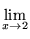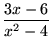,

we execute the Maple command
>
limit((3*x-6)/(x^2-4),x=2);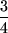Maple can also deal with limits which do not exist, for example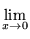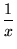:

>
limit(1/x,x=0);In the following exercise, we define the slope of a straight line passing through the points (x1, y1), (x2, y2), use this function to find the slope m(x) of the line passing through the points (1, 1) and (x, x2), and finally compute the limit of m(x) as x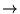1:

>
slope:=(x1,y1,x2,y2)->(y2-y1)/(x2-x1);
m:=x->slope(1,1,x,x^2);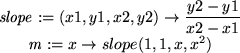>
limit(m(x),x=1);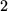Maple is sometimes unable to determine a limit or whether it exists. In such a case, it will return nothing after you execute the limit command.

Maple computes two-sided limits. For example, if you specify the argument x=1 as in the previous example, Maple assumes you mean that x approaches 1 from either the right or the left through real values only (as opposed to complex ones). However, Maple can compute one sided and complex limits also:

>
limit(1/x,x=0,right);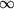>
limit(1/x,x=0,left);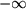>
limit(x*log(x),x=0,complex);You can also compute limits as x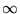and limits of functions of more than one variable:

>
limit(arctan(x),x=infinity);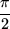>
limit(x/(x^2+y^2),x=0,y=0);A common error is to try to compute the limit of a function f(x) when x has been previously given a value. If you find yourself in this situation, unassign the value of x executing the command x:='x';. The previous example will fail if y still has the value assigned to it in section 4.2.Next: The diff (and Diff) Up: Preliminaries about Maple Previous: Assignments, Functions and Constants

Translated from LaTeX by Scott Sutherland
2002-08-29